# SMO forecast for SVM with polynomial kernel in Weka

This post shows a use in Weka of the SMOreg regressor (Sequential Minimal Optimization) which is an efficient machine learning algorithm for SVM (Support Vector Machine) to implement the approximators; SMOreg can be used also to implement predictions (forecast) on timeseries; the used kernel is a simple one, namely it is the PolyKernel (Polynomial Kernel) and the degree of polynomial will be 1. The objective is to demonstrate that classic machine learning forecast can achieve interesting levels of accuracy with extremely short learning times.
Although LSTM (Long Short Memory Term) type neural networks can be considered the best neural network typologies for the forecasting, a classic supervised machine learning forecast algorithm, like the one presented here, can reach acceptable levels of accuracy and with a significantly lower computational cost than that of an LSTM neural network.

In the real world datasets pre-exist the learning phase, in fact they are obtained by extracting data from production databases or Excel files, from the output of measuring instruments, from data-loggers connected to electronic sensors and so on, and then used for the following learning phases; but since the focus here is the forecast itself and not the prediction of a real phenomenon, the datasets used in this post have been synthetically generated from mathematical functions: this has the advantage of being able to stress the algorithm and see for which types of datasets the algorithm has acceptable accuracy and for which the algorithm is struggling.

## Complete sequence of steps

Launch the Weka program of the University of Waikato (New Zealand); in this post the version of Weka used is 3.8.3, but what I said also works with previous versions; Weka requires that the Java runtime is installed correctly.
After the Weka program is launched, the following window appears: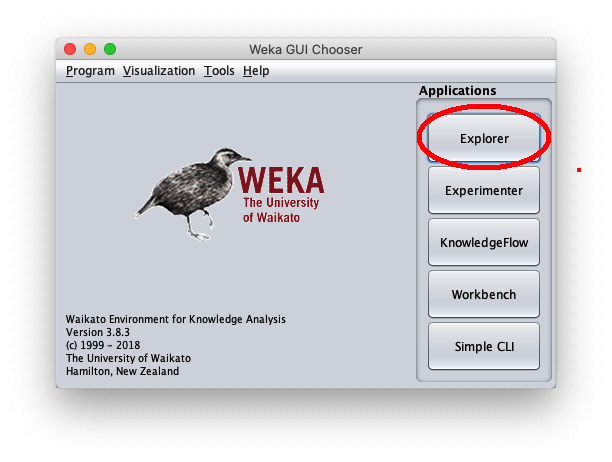press the Explorer button highlighted in red.
When the Weka Explorer window is displayed: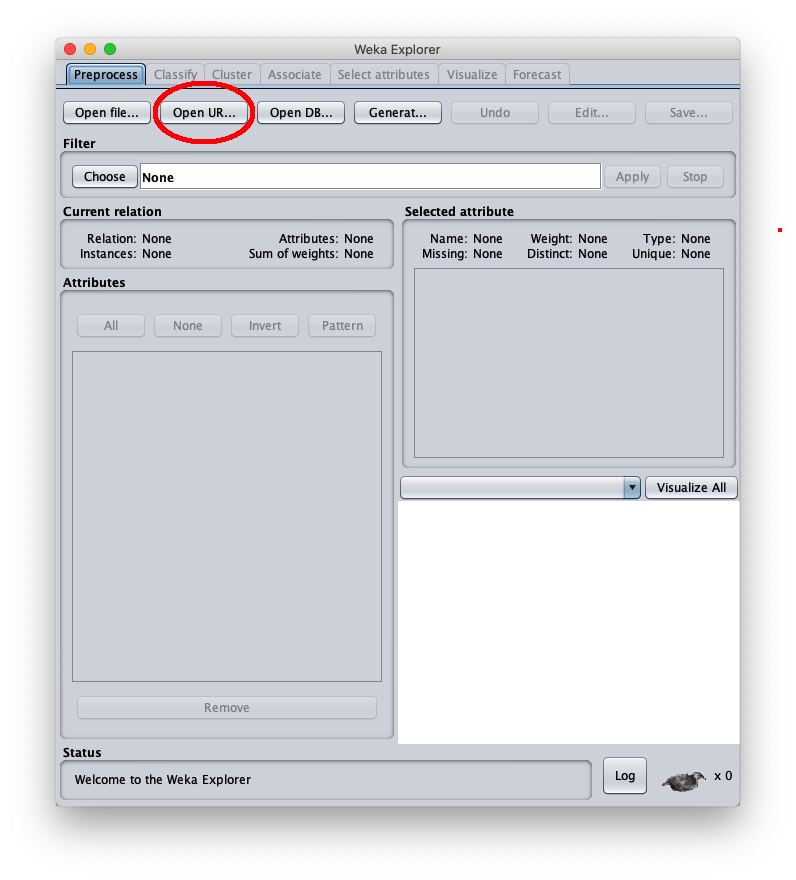press the Open Url... button, highlighted in red, to load the dataset in .arff format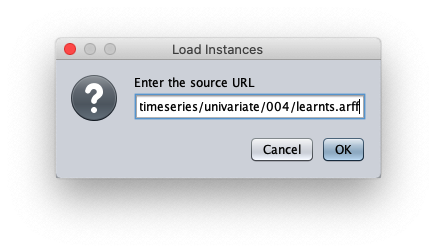paste the dataset url 004/learntss.arff and press Ok.
The loaded synthetic time series is generated with the formula $y=\frac{t}{50} + \sin \frac{t}{10}$ within $t \in I\!N, 0 \leq t < 200$;
the Weka Explorer window (located on the Preprocess tab) looks like this: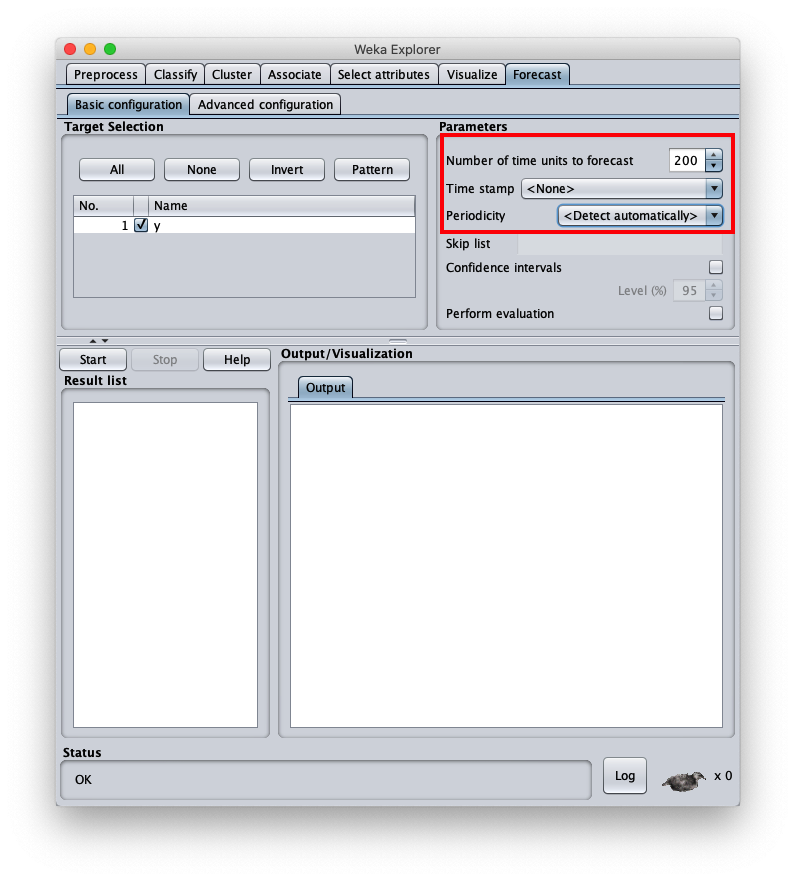For the purposes of the objectives of this post we can ignore this tab, then open the Forecast tab as highlighted in red and the user interface appears like this:and change Number of time units to forecast to 200 (the number of predictions to compute), Time stamp to None (because the chosen timeseries has no explicit field for time) and Periodicity set to Detect automatically (even though it would be known).
To choose the forecast algorithm in question, go to tab Advanced configuration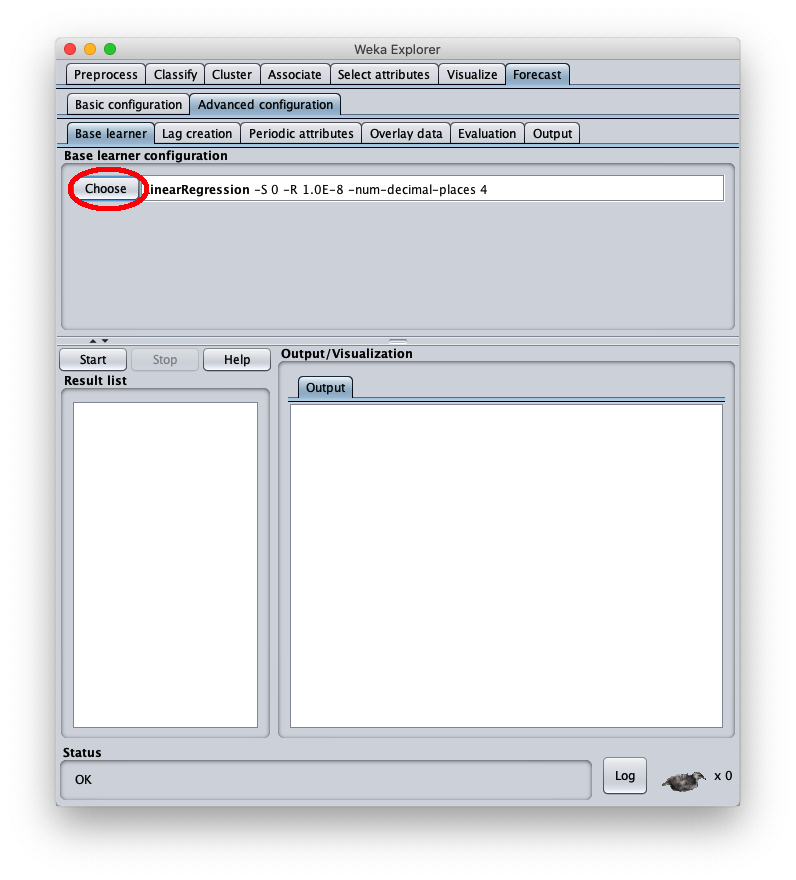and press the Choose button and this popup is shown on the screen: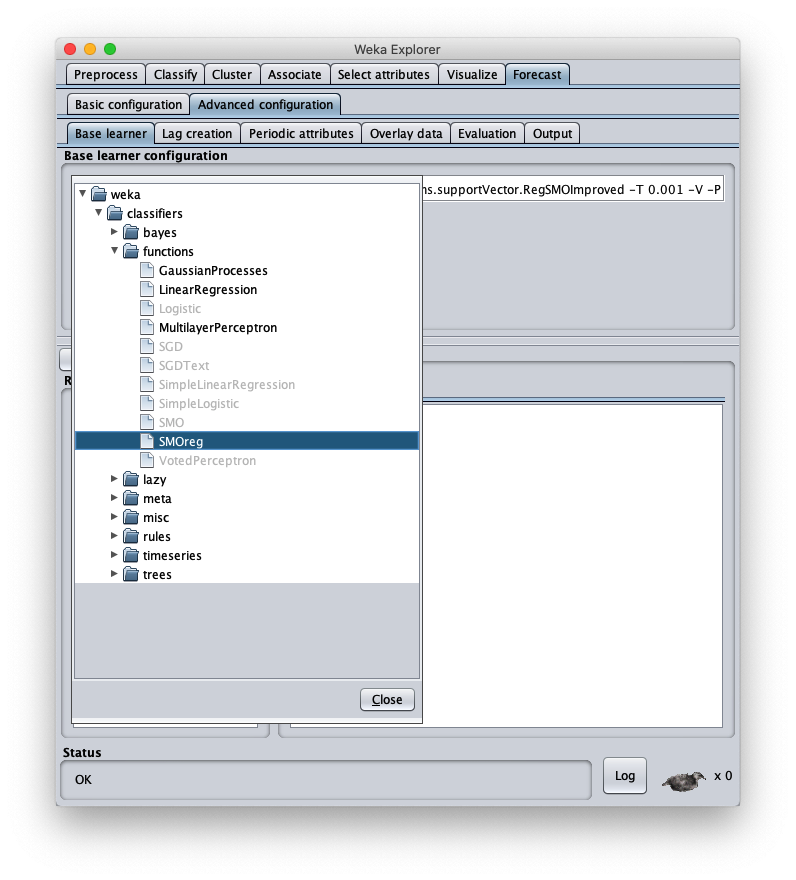and choose the SMOreg entry under the functions category; the user interface looks like this: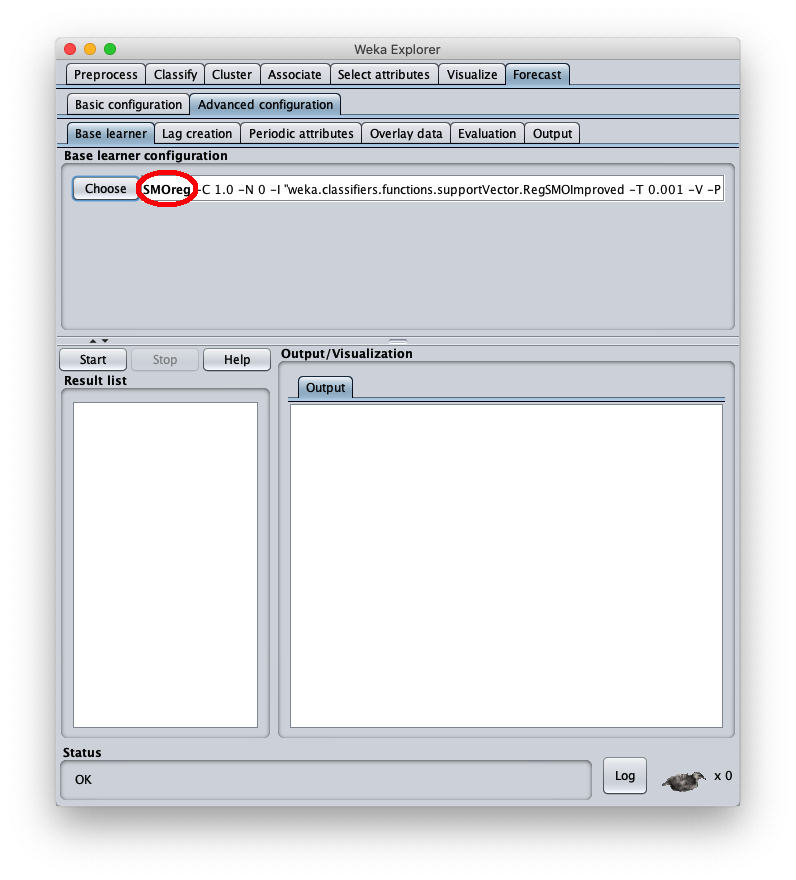Then press the SMOreg label to configure the parameters of the algorithm Sequential Minimal Optimization (abbreviated SMO):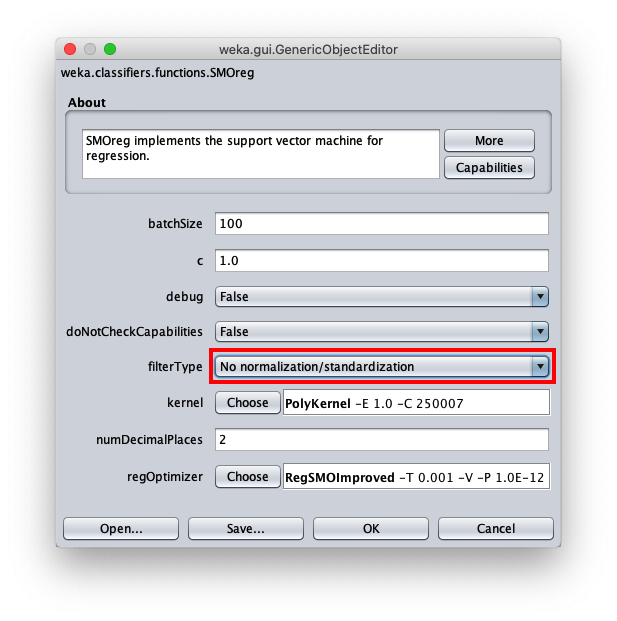Namely it is important to set the parameter filterType to No normalization/standadization; for all other parameters keep the default proposed values; then press OK.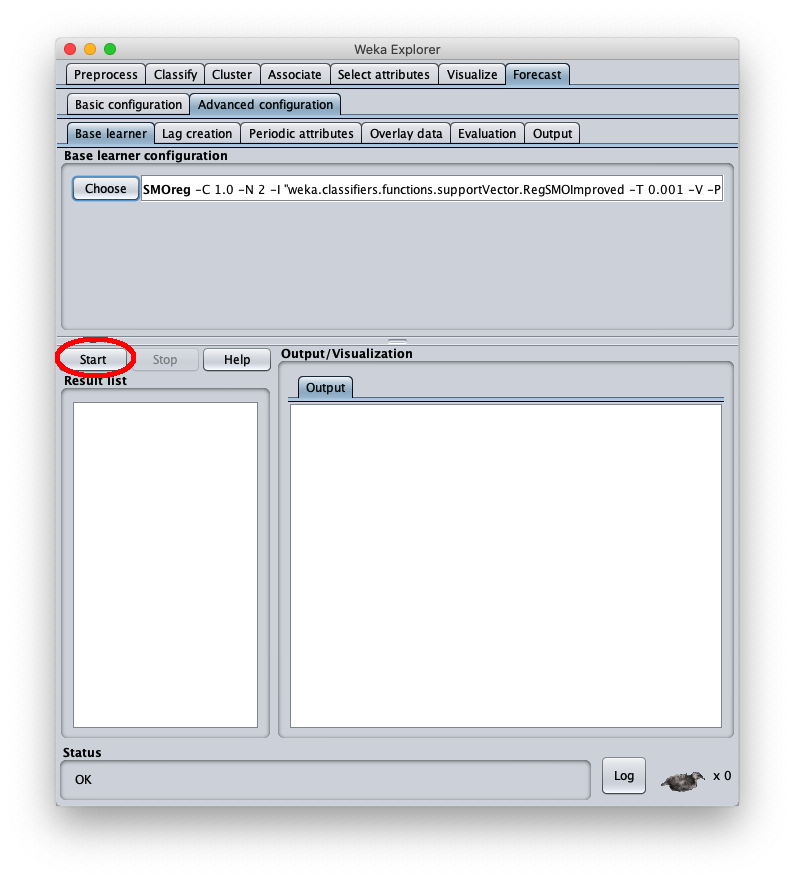Press the Start button to perform the forecast; after a few seconds of processing in the tab Train future pred. of the right part of the window Weka shows the graph of the timeseries of the dataset (abscissae from 0 to 200) and the prediction (abscissae over 200).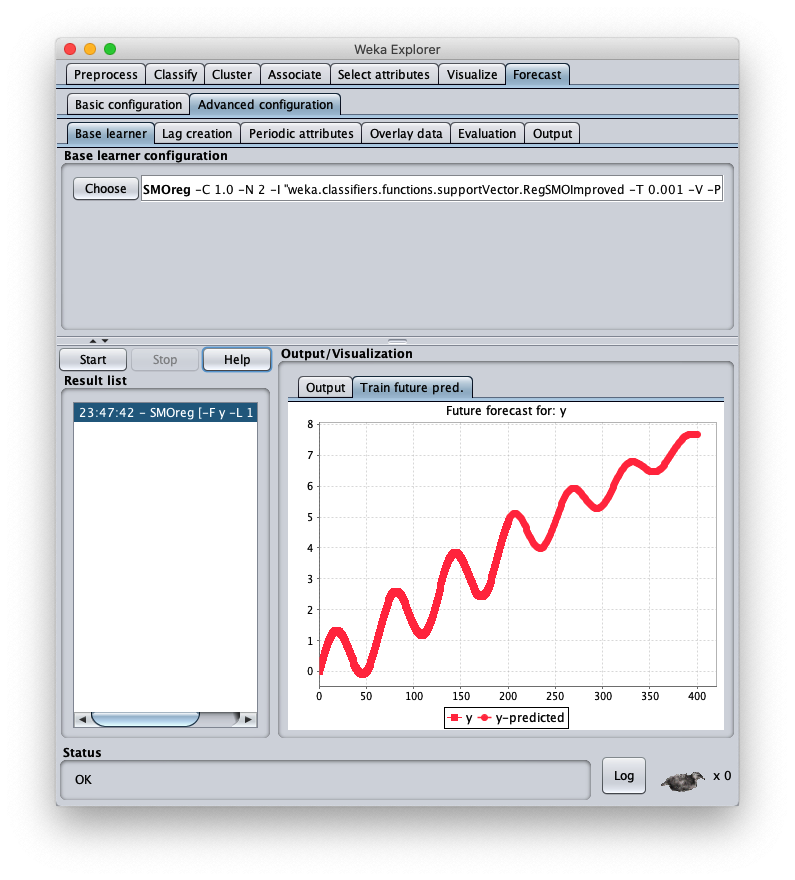Note that learning the trend is right and also the periodicity seems right, but it tends to crush the amplitude as the abscissae increases.
To correct this, open the parameters of the SMOReg algorithm configuration again: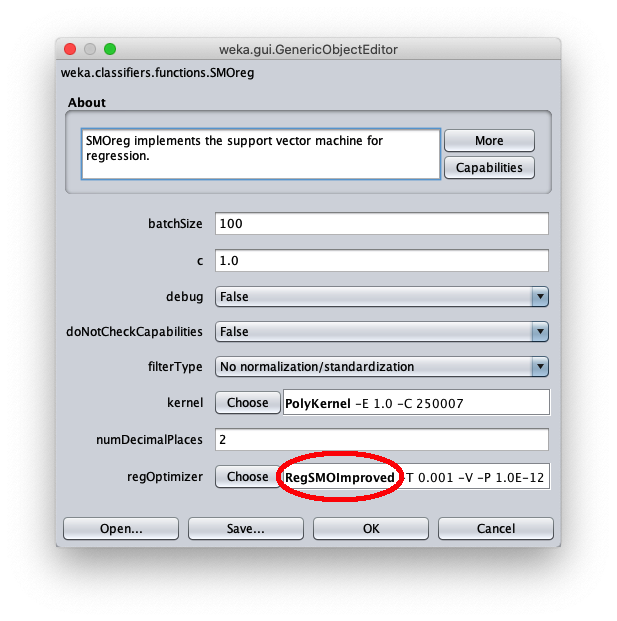click on the label RegSMOImproved as highlighted in red; the following popup è is shown: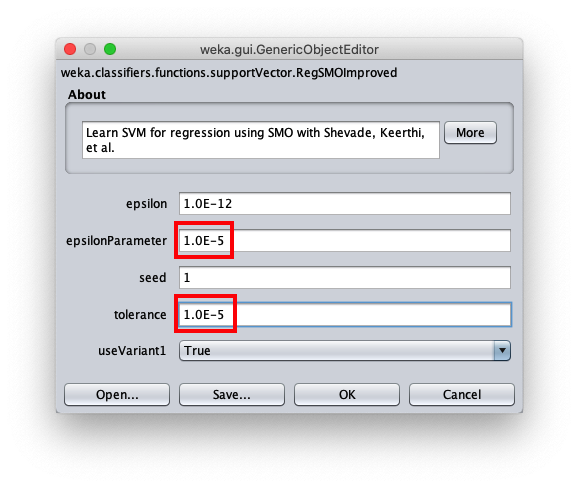set epsilonParameter and Tolerance parameters to 1.0E-5. Return to Weka Explorer by closing the two open popups with the OK button and press Start again to compute a new forecast.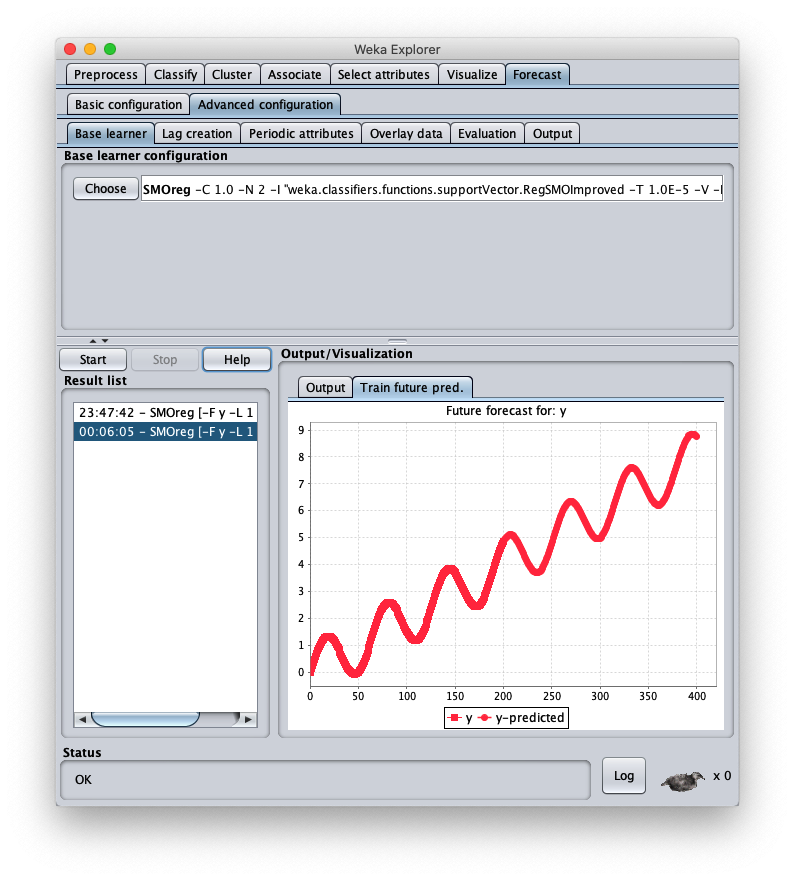As the graph clearly shows, the forecast obtained has the same periodic form and trend as the initial time series.